# Nuclear Reactivity Calculation with Reduction of Fluctuations

Title: Nuclear Reactivity Calculation with Reduction of Fluctuations
Daniel Suescun-Diaz, Geraldyne Ule-Duque, Jesus Antonio Chala-Casanova

Corresponding email:

Suescun-Diaz, D., Ule-Duque, G., Chala-Casanova, J.A., 2023. Nuclear Reactivity Calculation with Reduction of Fluctuations. International Journal of Technology. Volume 14(5), pp. 1113-1122

130
 Daniel Suescun-Diaz Department of Exact and Natural Sciences, Surcolombiana University, Neiva, 410001, Colombia Geraldyne Ule-Duque Department of Exact and Natural Sciences, Surcolombiana University, Neiva, 410001, Colombia Jesus Antonio Chala-Casanova Department of Exact and Natural Sciences, Surcolombiana University, Neiva, 410001, Colombia
Email to Corresponding Author

Abstract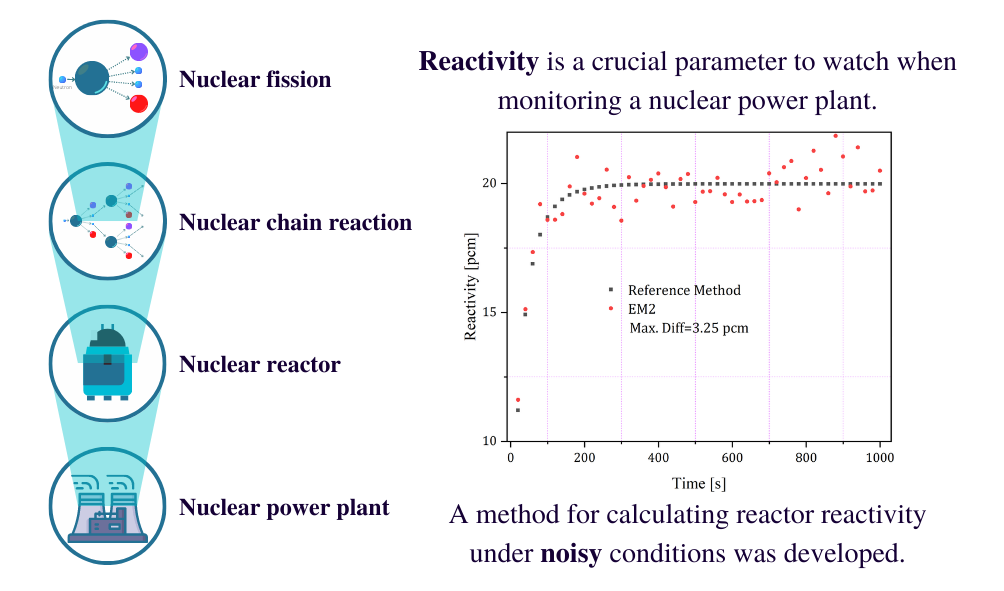This article presents a study based on a series of numerical experiments. It demonstrates the possibility of reducing fluctuations in the calculation of reactivity using the second Bernoulli number based on the approximation of the Euler-Maclaurin formula. This approach requires knowledge of the first three derivatives, which are implemented progressively. The fluctuations are assumed to occur around an average value of the neutron density with a Gaussian distribution. Jitter reduction is performed with a first-order delayed low-pass filter for different forms of neutron population density, with different time steps and with different filter constants. The numerical results show that the method can be used as a digital reactivity meter.

Inverse point kinetics equation; Neutron population density; Numerical experiment; reactivity; Second Bernoulli number

Introduction

The increasing world population has led to a higher demand for electrical energy in recent years. As a critical component of supporting a country's economic growth (Saroji et al., 2022), the electric power system plays a crucial role in meeting this demand. An alternative path would be to provide a solution where home automation systems can reduce electricity consumption (Rabbani and Foo, 2022). Another viable option is urban wind energy which is one of the new renewable ways of producing electricity; however, researchers have not studied very much in this field (Krasniqi, Dimitrieska, and Lajqi, 2022). Nuclear energy is another viable option obtained through nuclear reactors. However, it is necessary to know the reactivity parameters with good accuracy in nuclear reactors to operate a nuclear power plant more safely. Therefore, one of the main functions of a nuclear power plant is to control and monitor reactivity (Hyvarinen et al., 2022).

In recent decades, different experimental and computational methods have been developed to estimate the reactivity value in the core of a nuclear reactor. Studies have been carried out in a BAEC TRIGA Mark- II research reactor to analyze the effects of reactivity insertion, as well as in a prototype fast breeder reactor Monju (Hossain et al., 2022; Ohgama et al., 2022). The results are validated by a deterministic model given by the Inhour equation and the Monte Carlo method. The in-hour equation is also used in estimating reactivity in experiments conducted in the light water reactor at the VENUS-II experimental facility (Jiang et al., 2022).

Solving the inverse point kinetics equation provides a mathematical model that allows the calculation of reactivity. This method is commonly employed in computer-based simulations and facilitates the development of digital reactivity meters. To apply this model, the neutron density is required as an input, which can be measured using devices like ionization chambers (Vasilenko, Pankin, and Skvortsov, 2019). Studies that take this perspective are being conducted in the context of the decommissioning of the Fukushima Daiichi nuclear power plant. This includes a preliminary analysis that identifies the range that applies to the MIK code, such as ramped reactivity insertion (Fukuda, Nishiyama, and Obara, 2021).

A method has been developed to correct reactivity values by accounting for changes in both the neutron flux function and detector efficiency (Zhitarev et al., 2021). Based on an analysis of the inverse point kinetics equation, the influence of the background current on the measured reactivity is analyzed and a method for iterative calculation of reactivity is introduced (Huo et al., 2019). An accurate numerical solution for the inverse point kinetics equation is given using the discrete Fourier transform (Suescun-Diaz, Lozano-Parada, and Rasero-Causil, 2019). The extended Kalman filter technique (Bhatt et al., 2013) and the wavelet-based multiscale extended Kalman filter technique have also been proposed for reactivity estimation (Patel Mukhopadhyay, and Tiwari, 2018). However, reactivity meters based on the inverse point kinetics equation have sufficient capabilities to accurately estimate reactivity (Shimazu, 2014).

Due to gamma radiation, electronic system noise, and environmental radiation, there is considerable noise in the electrical current during reactivity measurement by external detectors, which leads to significant reactivity error, especially at low powers (Huo et al., 2019). Under these noise conditions, it is necessary to apply a filtering tool to smooth or reduce the fluctuations in the measurements.
In the present work, we study the approximation to solve the integral in the inverse point kinetic equation using the Euler-Maclaurin formula (Arfken, Weber, and Harris, 2013), which provides a powerful connection between integrals and sums, considering the approximation of the second Bernoulli number, with the combination using the first-order delayed low-pass filter to reduce fluctuations in the reactivity calculation. The results indicate that it is an alternative method for reactivity calculation with good approximation and can be used to design a digital reactivity meter.

Experimental Methods

2.1. Theoretical Considerations
The point kinetics equations are a set of differential equations that describe the time evolution of the expected values of the neutron density and the concentration of delayed neutron precursor groups in the core of a nuclear reactor. These equations accurately describe the reactor core dynamics and correspond to the time component of the neutron diffusion equation under the assumption of an isotropic and homogeneous medium (Stacey, 2018). They are described as follows: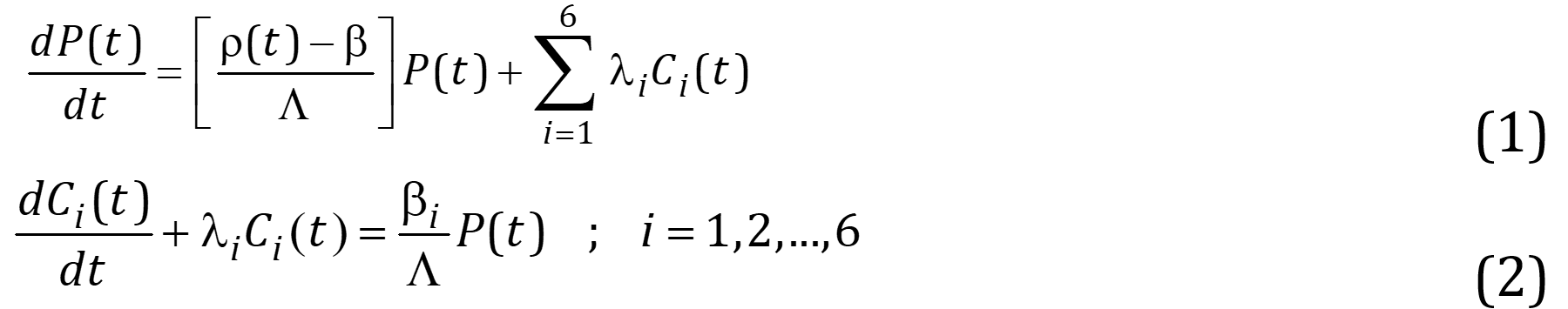The following initial conditions apply to equations (1) and (2),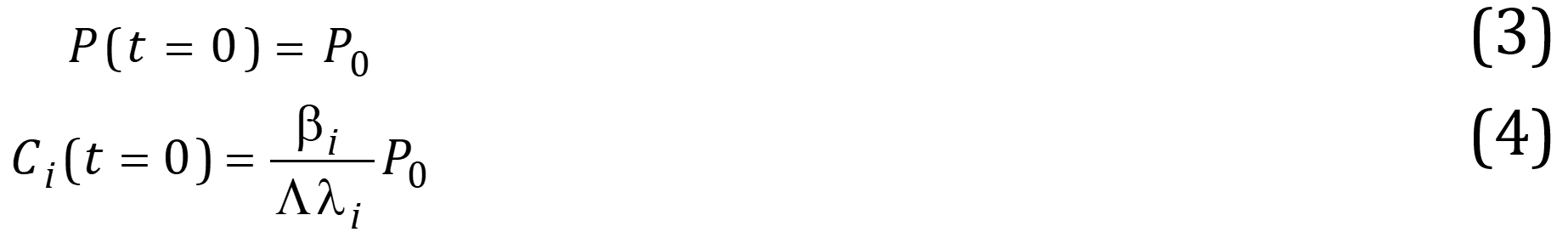where, P(t) is the neutron density, Ci(t) is the concentration of the i-th group of delayed neutron precursor,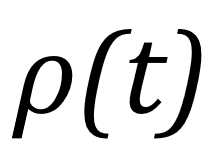is the reactivity,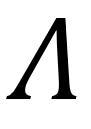is the neutron generation time,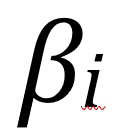is the i-th fraction of delayed neutrons,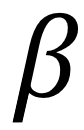is the total effective fraction of delayed neutrons,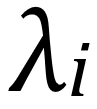is the decay constant of the i-th group of delayed neutron precursors.
Solving forfrom equation (1) leads to the following reactivity equation: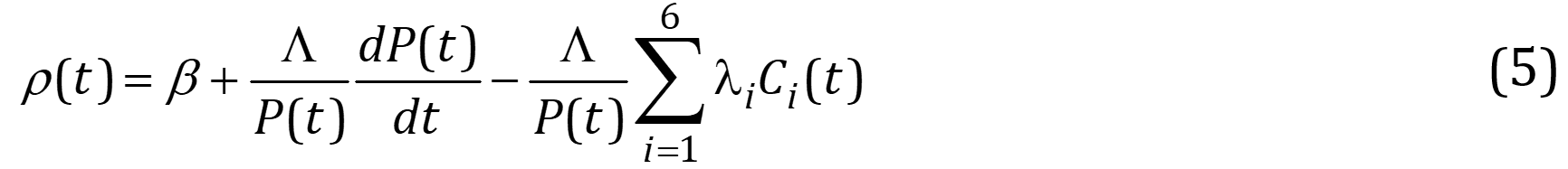The unknown term in equation (5) is the concentration of precursors Ci(t), which can be found by solving equation (2) by an integrating factor or by the Laplace transform -considering equations (3) and (4)- will lead to the following expression: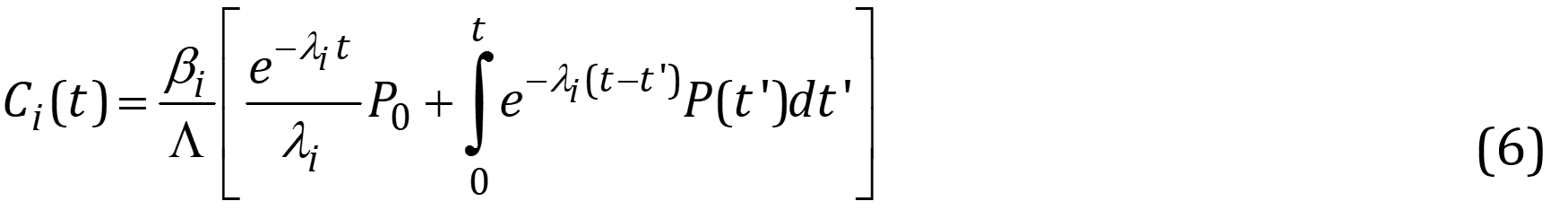By replacing equation (6) with equation (5), a new equation for reactivity is obtained, which needs all the values of the neutron density to be known: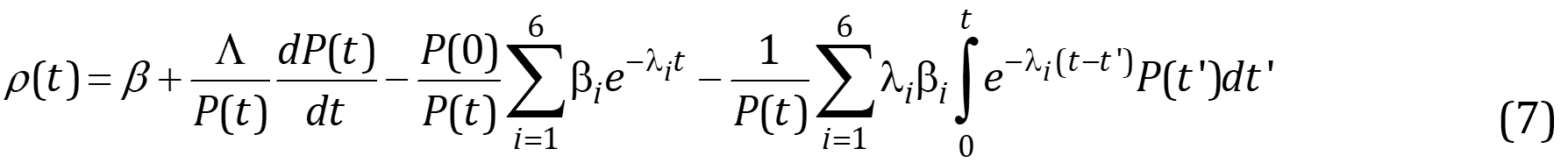Equation (7) is the so-called inverse point kinetic equation and allows the calculation of the nuclear reactivity, which provides information on the behavior of a reactor core. This equation has been a model that has been applied in the design of digital reactivity meters that contribute significantly to the control and safe operation of a nuclear reactor. However, its dependence on all the values of the neutron density in a non-recursive way causes a high computational cost, which makes it difficult to calculate the reactivity in real-time. To reduce the computational cost, the following section presents a method that discretizes the integral containing the dependence on the neutron density by using the Euler-Maclaurin formula with an approximation of two Bernoulli numbers.

2.2. Proposed Method

To achieve greater accuracy in reactivity calculations while minimizing computational costs, it is necessary to discretize the integral term in equation (7). This is accomplished by applying the Euler-Maclaurin formula as follows (Kwok, 2010):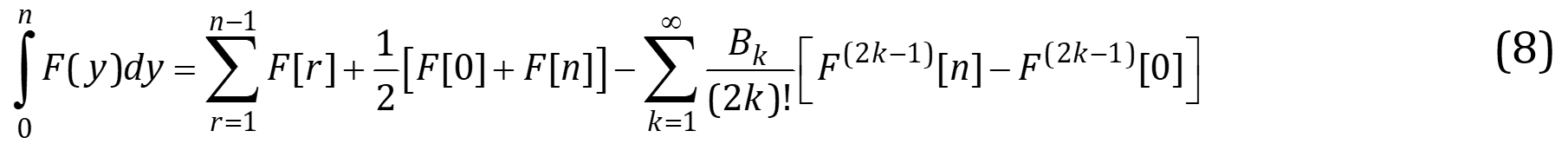where the term Bk represents the Bernoulli numbers.

Substituting equation (8) into equation (7), reactivity with the approximation of the first Bernoulli number B1=1/6 is obtained (Suescun-Diaz, Ule-Duque, and Pena-Lara, 2020). To find a descriptive expression for reactivity with the approximation of the second Bernoulli number B2=1/30, substitute k=2 into equation (8), taking derivative three times leads to: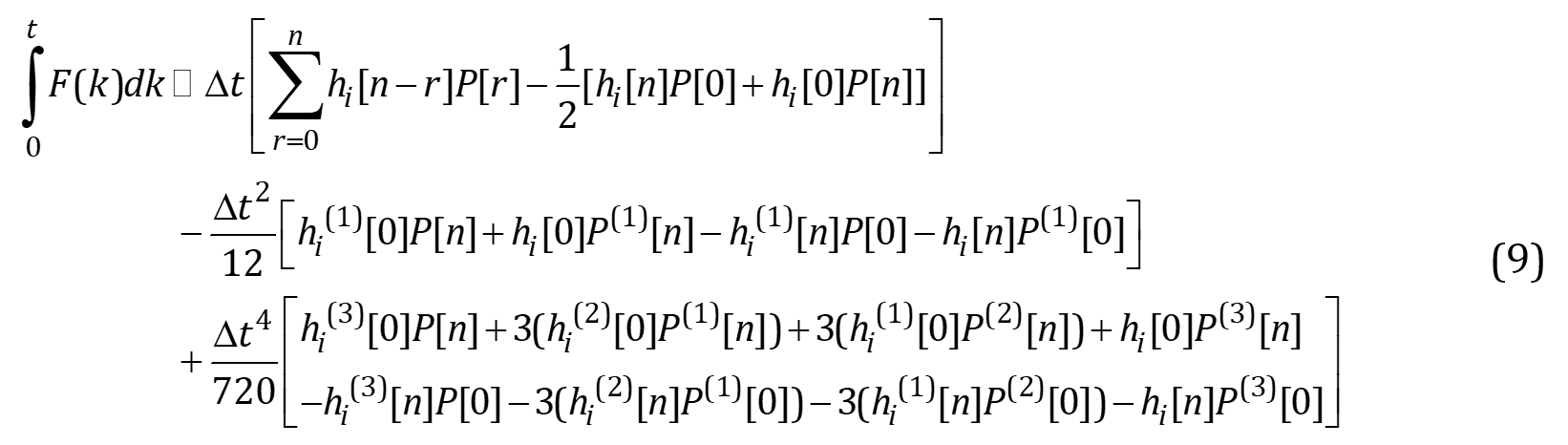Being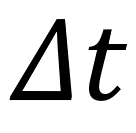the time step in the reactivity computation, n indicates the discrete-time, and its relation to the continuous time is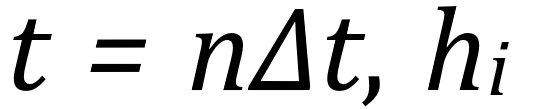is the system response to a unit impulse function (Haykin, 2014) defined here as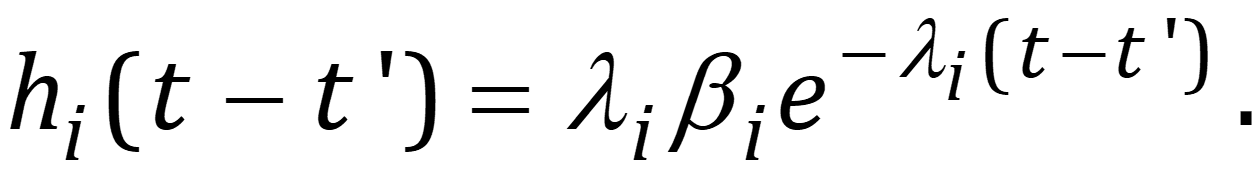Replacing equation (9) into equation (7), the following expression for reactivity is obtained: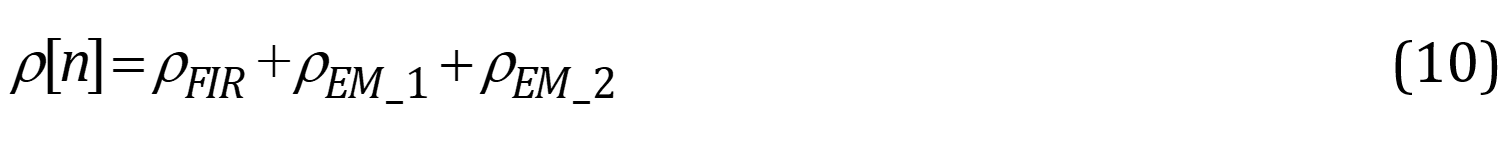Where,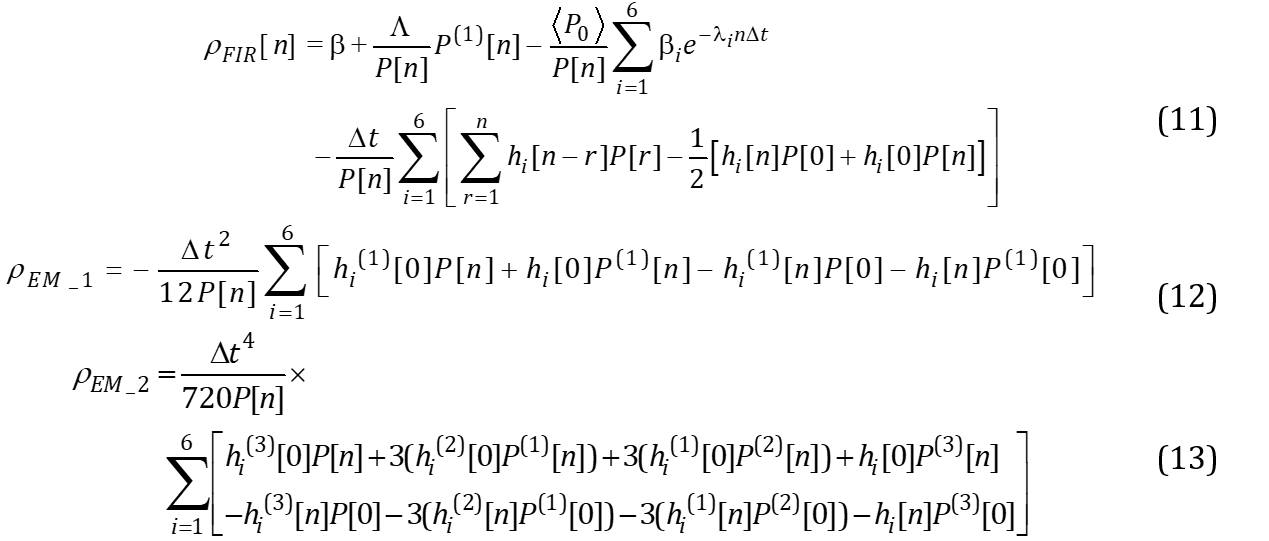Equation (10) represents the reactivity with the approximation of the first two Bernoulli numbers, being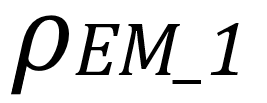the correction of the first Bernoulli number represented in equation (12), and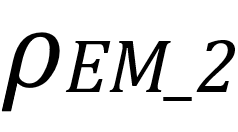the correction with the second Bernoulli number represented in the equation (13).

For the calculation of the first, second and third derivatives, the progressive derivatives are implemented (Mathews and Fink, 2005) as given by equations (14-16):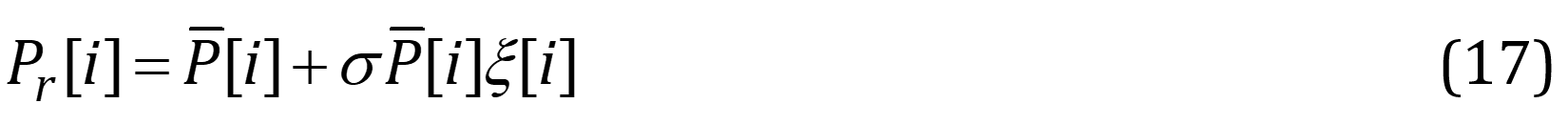where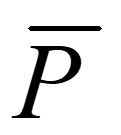is the average value of an arbitrary deterministic signal,is a Gaussian noise,is the standard deviation and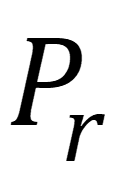will be the new signal containing fluctuations. To reduce the fluctuations in the calculation of reactivity, a low-pass filter with first-order delay is considered here (Tamura, 2003; Kitano et al., 2000) which is given by the expression: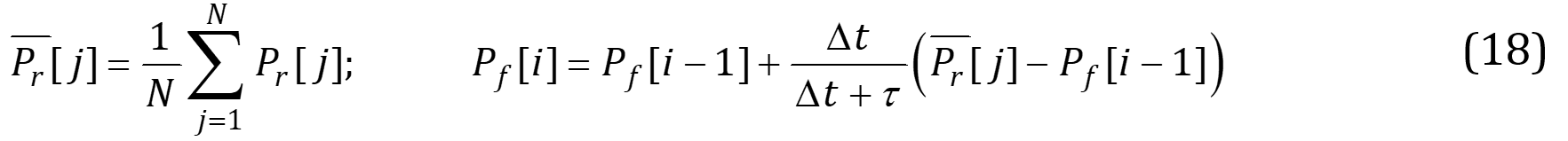Whereis the filtering constant.

There are 422 nuclear reactors in operation and another 56 under construction with 377 872 MWe and 58 584 MWe total net installed capacity, respectively. The reactivity value is critical for ensuring the safe operation of nuclear reactors. Therefore, the objective of the proposed method in this work is to numerically solve the inverse point kinetics equation using equation (10). The low-pass filter given by equation (18) is proposed to reduce the fluctuations of an input signal associated with neutron population density measurements.

The simulations were implemented using the MATLAB computational tool. The physical constants used in this work are due to the interaction of neutrons with the combustible material 235U. These constants are the decay constant= [0.0127, 0.0317, 0.115, 0.311, 1.4, 3.87]s-1, the delayed neutron fraction= [0.000266, 0.001491, 0.001316, 0.002849, 0.000896, 0.000182], the total delayed neutron fraction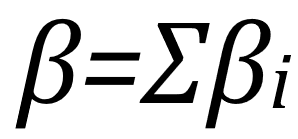and the instantaneous neutron generation time=2×105s (Ganapol, 2013). For noise simulation, a seed generating random numbers of 231-1 is used. Some results of the different numerical experiments for calculating reactivity are presented in the next section. Consider different forms of neutron density, time steps and filter constants.

Results and Discussion

The physical parameters in this results section are considered as above for a thermal reactor with 235U fuel elements. The most outstanding results obtained for different numerical experiments are presented using the proposed method given by equation (10) and denoted by  when considering noise in the neutron density and EMF2 when filtering this noise using the low-pass filter given by equation (18). Initially, the shape of the neutron density is considered exponential, which is found in the design of a nuclear reactor. The different numerical experiments are performed for the neutron density of the form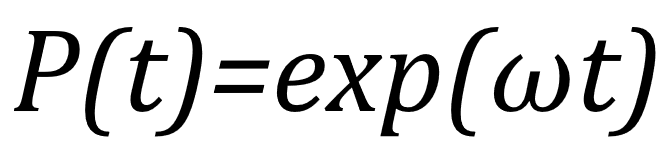with different values of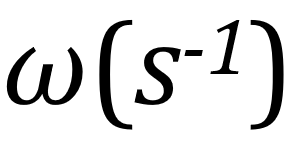obtained from the inhour equation . Finally, the neutron population of form P(t)=a+bt3 is considered with different values of b. The reference method is obtained by the analytical solution of equation (7), which allows an analysis of the accuracy of the method.

It is necessary to know the reactivity with high precision; however, in practice, the neutron population density contains noise, which has a Gaussian distribution that produces fluctuations or uncertainties in the reactivity calculation. To reduce fluctuations, the first-order delay low-pass filter given by equation (18) with a filtering constant of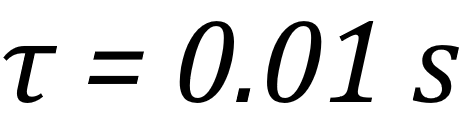and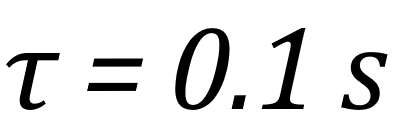is used. In all numerical experiments, the time step varies in the interval [0.001, 0.1] s, and the standard deviation is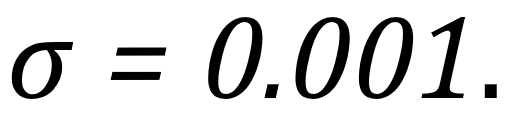For the different derivatives of neutron density represented in equations (11-13), the progressive derivatives are taken (Mathews and Fink, 2005), represented by equations (14-16).

Table 1 shows the maximum differences in reactivity in pcm (parts per hundred thousand) for a neutron density of the form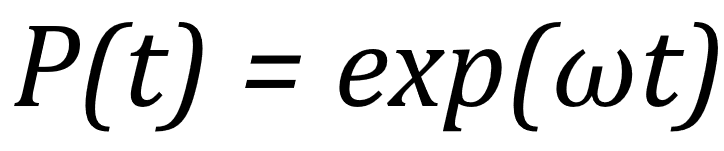with a value of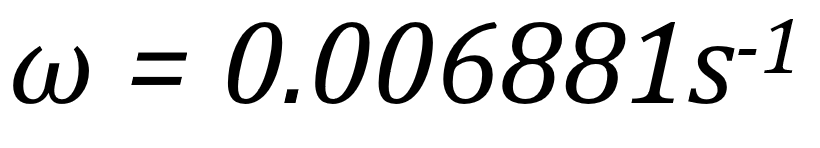obtained from the inhour equation that provides a reactivity of about 50 pcm. It is possible to observe that for this reactivity value, the reduction of the fluctuations (RF) is effective for any time step, obtaining a reduction above 50% for any case, although the most significant occurs when a constant filter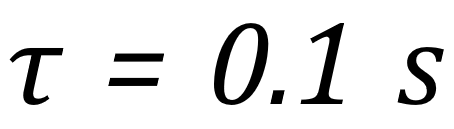and a time step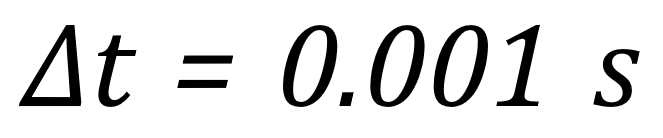are used, reaching a reduction of 84.06%. This significant reduction indicates that the uncertainty in the reactivity value decreases. In other words, it increases the precision in the calculation of the reactivity that would achieve greater control of the reactor. This RF is calculated as follow:  This RF is calculated as shown in equation (19).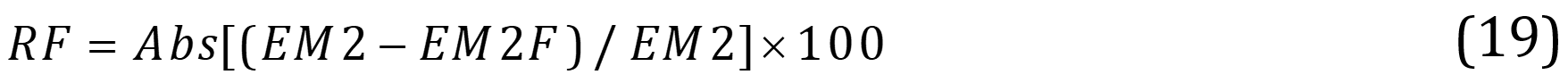To validate the results obtained with the proposed method for the exponential form of neutron density, a time-step of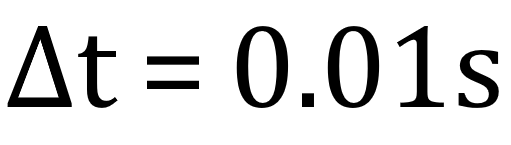is considered for the EM2F case. The results show a maximum difference of 0.88 pcm. In a study by Suescun-Díaz, Lozano-Parada, and Rasero-Causil (2019) under the same conditions, a maximum difference of 2.06 pcm was reported using the discrete Fourier transform. These results clearly demonstrate that the EM2F method yields the highest level of reduction.

Table 1 Maximum difference in reactivity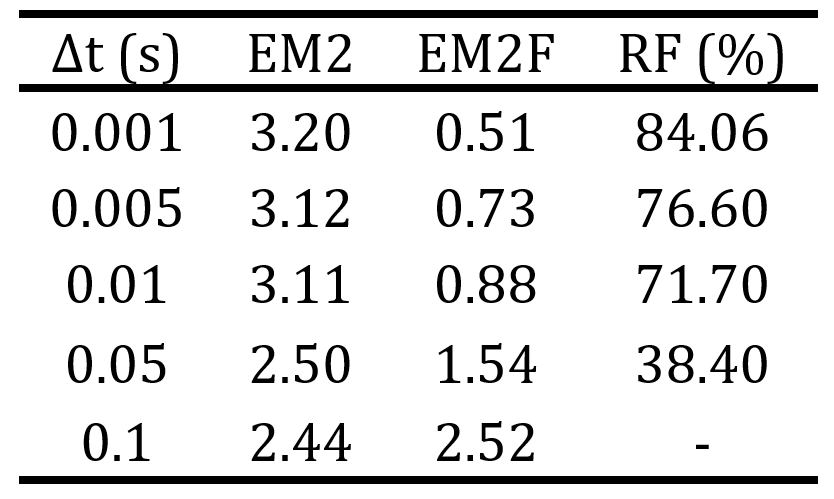In order to be able to make decisions in the operation of a nuclear reactor using control rods, the reduction of fluctuations is presented in Figures 1 and 2, the reactivity for a form of neutron densitywith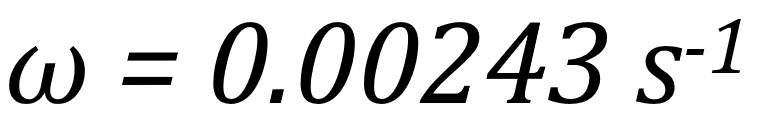andwithout low-pass filter (EM2) and with a low-pass filter (EM2F) at a filter constant of, respectively. It can be observed that when a first-order low-pass filter is applied, the fluctuations are effectively reduced.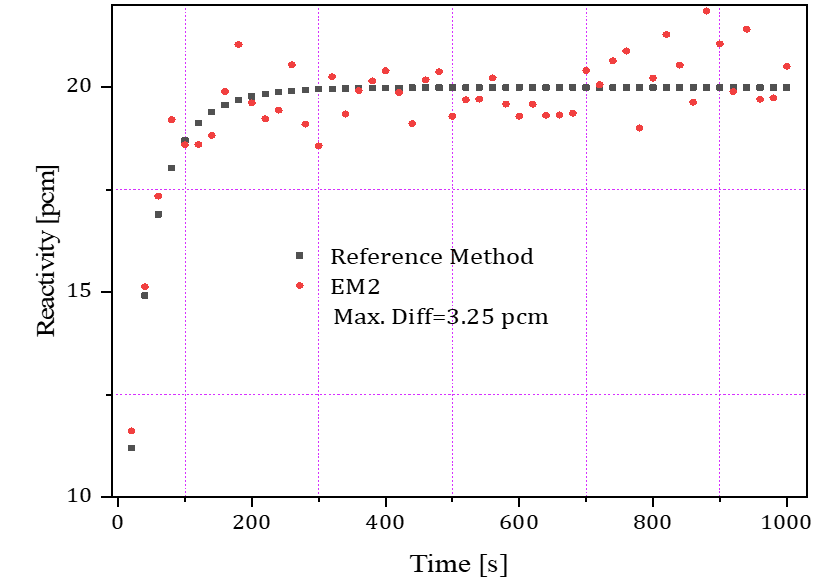Figure 1 Reactivity for a neutron densitywithout low-pass filter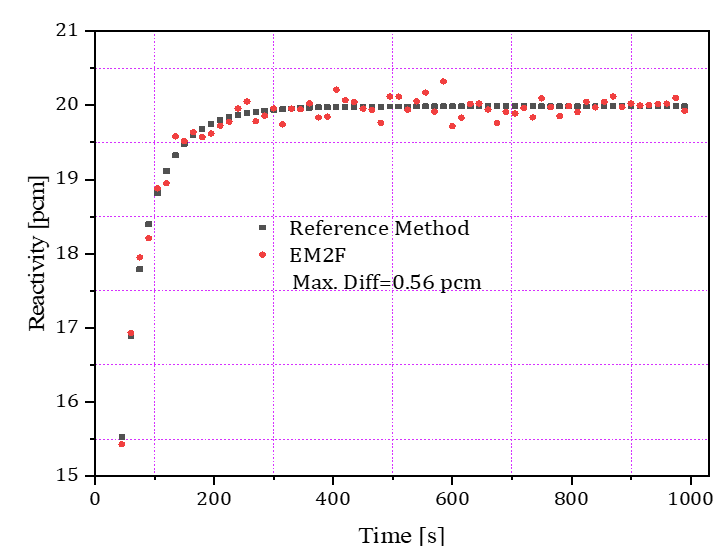Figure 2 Reactivity for a neutron densitywithwith low-pass filter

Table 2 considers the neutron density of the form, which gives a reactivity of about 20 pcm. It is evident that for time steps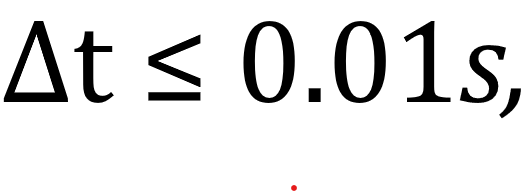the reduction of fluctuations with the proposed method is significant. The achieved reduction ranges from 56.64 % to 92.17 %. In this numerical experiment, it was found that the fluctuations are reduced even for a time step, reaching a minimum reduction of 56.64 %.

Table 2 Maximum difference in reactivity